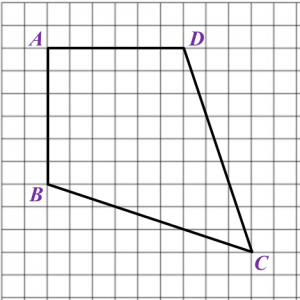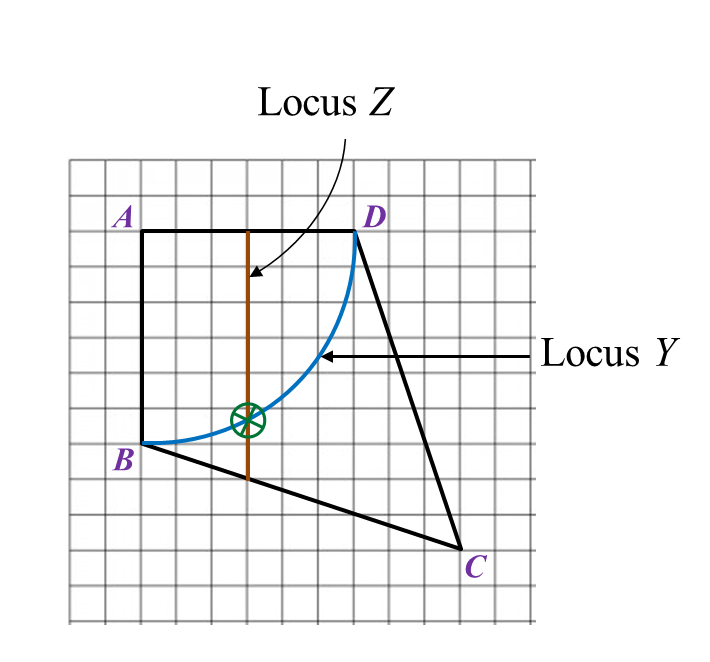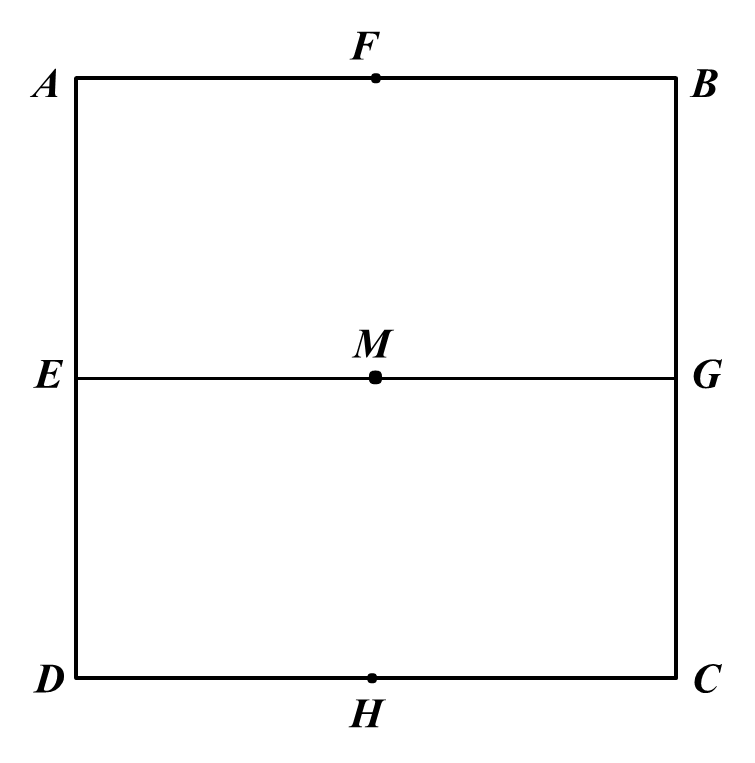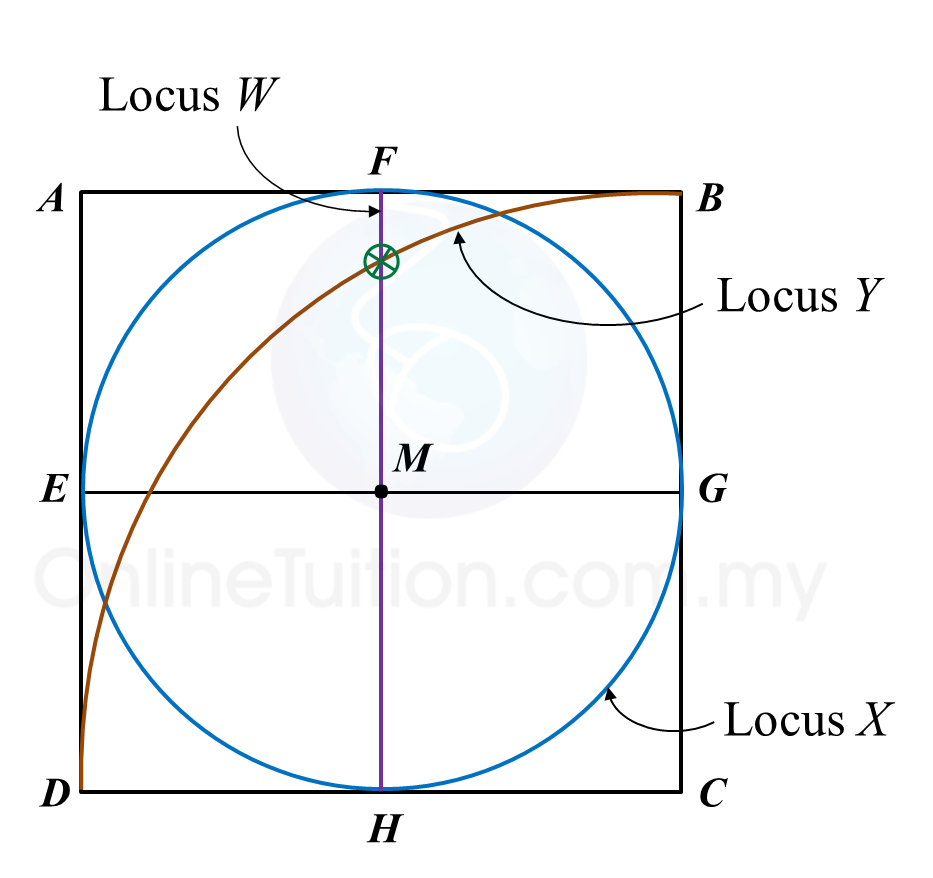# 8.2.2 Loci in Two Dimensions, PT3 Focus Practice

 
        
       
8.2.2 Loci in Two Dimensions, PT3 Focus Practice
       

 Question 3:
       
Diagram below in the answer space shows a quadrilateral ABCD drawn on a grid of equal squares with sides of 1 unit.
       
X, Y and Z are three moving points inside the quadrilateral ABCD.
 
 
       
(a) X is the point which moves such that it is always equidistant from point B and point D.
       
By using the letters in diagram, state the locus of X.
 
 
       
(b) On the diagram, draw,
 
(i) the locus of the point Y such that it is always 6 units from point A,
 
(ii) the locus of the point Z which moves such that its distance is constantly 3 units from the
  line AB.
 
 
       
(c) Hence, mark with the symbol ⊗ the intersection of the locus of Y and the locus of Z.
       
       

 Answer
:
       
(b)(i),(ii) and (c)Solution
:
       
(a) The locus of X is the line AC.
       
       

 (b)(i),(ii) and (c)Question 4:
       
Diagram in the answer space below shows a square ABCD. E, F, G and H are the midpoints of straight lines AD, AB, BC and CD respectively. W, X and Y are moving points in the square.
       
On the diagram,
 
 
       
(a) draw the locus of the point W which moves such that it is always equidistant from point AD and BC.
 
 
       
(b)   draw the locus of the point X which moves such that XM = MG.
 
 
       
(c) draw the locus of point Y which moves such that its distance is constantly 6 cm from point C.
 
 
       
(d)   Hence, mark with the symbol ⊗  the intersection of the locus of W and the locus of Y.
       
       

 Answer
:
       
(a), (b), (c) and (d)Solution
: